# Interview questions on bitwise operators in cPreviously I have written an article “embedded c interview questions” that is liked by many professional and students. Nowadays I am getting many emails from readers and they request to me to create the list of some popular tricky questions on a bitwise operator. So here I have collected some popular bitwise operators questions that generally asked in the interview.

### Compute the sign of an integer?

The MSB bit of a number defines their sign. If the MSB bit is set, the number will be negative.

### Detect if two integers have opposite signs?

The two integer have the different signs if their MSB is different. In “C” Language using the EX-OR operator, we can check the sign of the integers.

We know that for the same input EX-OR produces the low output and for the different input it produces the high output.

E.g.

 BIT1 BIT2 BIT1  ^  BIT2 1 1 0 0 0 0 1 0 1 0 1 1

Let the given integers are “a” and “b”. The EX-OR of sign bit (MSB) of “a” and “b” will be 1 if the MSB of “a” and “b” is different.In other words, we can say, EX-OR of “a” and “b” will be negative if “a” and “b” have the opposite signs.

### Write a program to check an integer is a power of 2?

Here, I am writing a small algorithm to check the power of 2. If a number is a power of 2, the flag will be 1.

Note: Here I assume that bit of register starts with 0th position, it means the 2nd position is actually 3rd bits.

 D7 D6 D5 D4 D3 D2 D1 D0

### How to set a particular bit in C?

#### Setting a Bits

Bitwise OR operator (|) use to set a bit of integral data type.”OR” of two bits is always one if any one of them is one.

An algorithm to set the bits

Number  | =  (1<< nth Position)

#### Simple program to set a bit.### How to clear a particular bit in C?

Bitwise AND operator (&) use to clear a bit of integral data type. “AND” of two bits is always zero if any one of them is zero.

An algorithm to clear the bits

Number  &=  ~ (1<< nth Position)
To clear the nth bit, first, you need to invert the string of bits then AND it with the number.

### How to check if a particular bit is set in C?

To check the nth bit, shift the ‘1’ nth position toward the left and then “AND” it with the number.

An algorithm to check the bits

Bit = Number & (1 << nth)

### How to toggle a particular bit in C?

Bitwise XOR (^) operator use to toggle the bit of an integral data type. To toggle the nth bit shift the ‘1’ nth position toward the left and “XOR” it.

An algorithm to toggle the bits

Number  ^=  (1<< nth Position)

### Write an Efficient C Program to Reverse Bits of a Number?

There are a lot of ways to reverse the bits of a number, here I am describing three general methods to reverse the bits.

#### Method 1:

In this method, we will check the set bits of num and run the loop through all the bits of an integer.If we find the ith bits of num is set then just put 1 at the ((INT_BITS – 1) – ith ) position of tmp, where INT_BITS is the number of bits of an integer.

#### Method 2:

It is a simple algorithm to reverse bits of the 32-bit integer. This algorithm uses the eight constant value for the reversing the bits and takes five simple steps.

In below section, I am describing the functioning of each step.

Steps 1:
num = (((num & 0xaaaaaaaa) >> 1) | ((num & 0x55555555) << 1));

This expression used to swap the bits.
Let an example, suppose num is 0100, after the above expression it will be 1000.

Steps 2:
num = (((num & 0xcccccccc) >> 2) | ((num & 0x33333333) << 2));

Above expression uses to swap the 2 bits of a nibble. Suppose num is 10 00, after the above expression, it will be 00 01.

Steps 3:
num = (((num & 0xf0f0f0f0) >> 4) | ((num & 0x0f0f0f0f) << 4));

An expression used to swaps the nibbles. like if num is 0011 0010 then after the above expression it will be 0010 0011.

Steps 4:
num = (((num & 0xff00ff00) >> 8) | ((num & 0x00ff00ff) << 8));

This statement uses to swap the bytes of an integer. Let num is 00001000 00001100, after the above expression, it will be 00001100 00001000.

Steps 5:
((num >> 16) | (num << 16));

The above expression uses to swap the half-word of an integer. Means that if the num is 0000000011001110 1000100100000110 after the above result number will be 1000100100000110 0000000011001110.

### Write a program to count set bits in an integer?

There are a lot of ways to count the number of bits in a given integer, here I am writing two approaches naive and  Brian Kernighan’s.

In naive approach requires one iteration per bit until no more bits are set.

Brian Kernighan’s method goes through as many iterations as there are set bits.

1. Initialize CountSetBits = 0

2. If integer n is not zero.

( a ). Perform bitwise operation and assign the value back to the n.
These bitwise operations clear the least significant.
n &= (n – 1);

( b ). Increment CountSetBits by 1.

( c ). Again go to the step 2.

3. If there are no set bits remaining then return CountSetBits.

##### If you want to learn more about the c language, here 10 Free days C video course for you.### Rotate bits of a number in C?

Like the assembly in C language there is no operator to rotate the bits, so if we require rotating a bit, then we have to do it manually.

Basically, bit rotation is similar to the shift operation except that in shift operation the bits that fall off at one end are put back to the other end.

There is two type of rotation possible left and right. In the left rotation, the bits that fall off at left end are put back at right end and in right rotation, the bits that fall off at right end are put back at the left end.

Example:
If data is stored using 8 bits, then left rotation of a data 32(‭‬00100000‬) by 2 becomes ‭128 (10000000). As similar to left rotation, if data is stored using 8 bits, then right rotation of the data 32(‭‬00100000‬) by 2 becomes 8 (00001000).

### Compute the minimum (min) or maximum (max) of two integers without branching?

We can find the minimum (min) or maximum (max) number without the branching with the help of a bitwise operator.

Let’s assume “a” and “b” are integers numbers and “result” is another integer variable that contains the result of the
computation.

So to compute the minimum number we have to write the below expression.

Compute the maximum number we have to write the below expression.

### Swap two numbers without using a temporary variable?

Using EX-OR operator, we can swap two number. Here the concept is that EX-OR of two same number is zero.

### Clear all bits from MSB to ith bit

Here I have supposed data is stored using 8 bits.

let’s assume the ith position is 2.

mask =(1 <<( i+1)); // give you 00001000

so now if we subtract 1 from the mask (mask = mask – 1), then we will get 00000111

Using the mask, now we can clear MSB to ith bits of data (15).

data = data & mask; // Now bits are clear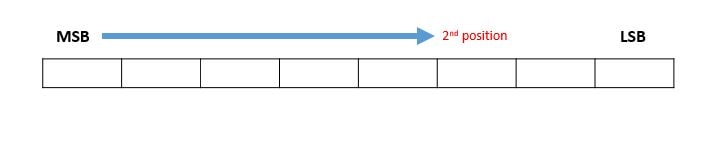### Clear all bits from LSB to ith bit

To clear all bits of a data from LSB to the ith bit, we have to perform AND operation between data and mask (flag) having LSB to ith bit 0.

To create a mask, first left shift 1 (i+1) times.

mask =(1 << (i+1)); // give you 00001000

Now if we minus 1 from that, all the bits from 0 to i become 1 and remaining bits become 0.

mask = mask – 1 ; // give you 00000111

After that perform complement operation on the mask, all the bits from 0 to i become 0 and remaining bits become 1.

Now just simply perform anding operation between mask and data to get the desired result.

data = data & mask; // Now bits are clear from LSB to ith position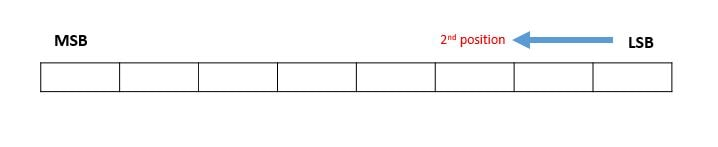### Multiply a number by 2 using bitwise operation

Left shifting of a data (number) by 1 is equivalent to data*2. In data, every bit is a power of 2, with each shift we are increasing the value of each bit by a factor of 2.

### Divide a number by 2 using bitwise operation

Right shifting of a data (number) by 1 is equivalent to data/2. In data, every bit is a power of 2, with each right shift we are reducing the value of each bit by a factor of 2.

### Multiply a given Integer with 3.5 using bitwise operation

We know that multiplication is a type of addition, so we can multiply a given integer (data) with 3.5 using the following operation, (2 *data) + data + (data/2).

### How to change endianness?

In below image, you can see the conversion.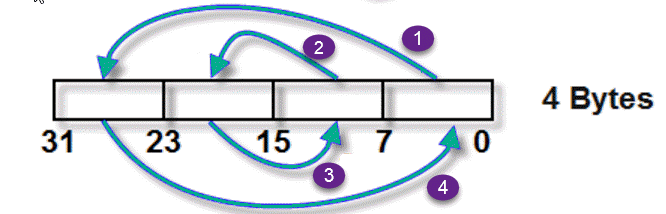##### Aticleworld invites you to try skillshare (Unlimited Access to over 20,000 classes) Premium free for 2 months.### Swap two nibbles of a byte

A nibble consists four bits, sometime interviewer asked the question to swap the nibble of a byte.It is a very easy question, here << (left shift) and >> (right shift) operators are used to swap the nibble.

### How do I get a bit from an integer value in C?

To get the ith bit, perform Anding operation between the ith bit and 1 (1 << i) after that shift the result i position in right using right operation.

### Write the macros to set, clear, toggle and check the bit of a given integer

See the below macro,

• #define SET_BIT(value, pos) value |= (1U<< pos)
• #define CLEAR_BIT(value, pos) value &= ~(1U<< pos)
• #define TOGGLE_BIT(value, pos) value ^= (1U<< pos)
• #define CHECK_BIT_IS_SET_OR_NOT(value, pos) value & (1U<< pos)

Let see an example to set the bit using the above macro,

Output: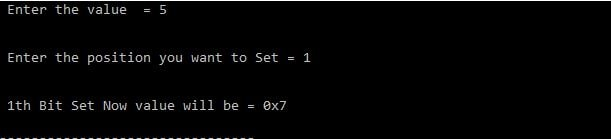### Write MACRO to swap the bytes in 32bit Integer Variable.

I have already written this program in endianness conversion. But here I am creating a Macro for the same.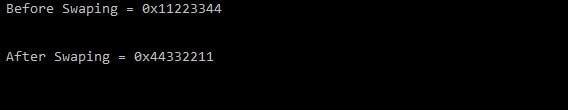### Swap all odd and even bits

In the above question, you need to swap the even and odd bits. To accomplish the above task you need to first find the even and odd bits then shift these bits. See the below steps,

Let the input number is data (Assuming integer size is 4 bytes),

1. Get all even bits of data by doing bitwise and (&) of data with 0xAAAAAAAA (data & 0xAAAAAAAA).
2. Get all odd bits of data by doing bitwise and (&) of data with 0x55555555 (data & 0x55555555).
3. Right shift all even bits ((data & 0xAAAAAAAA)>>1).
4. Left shift all odd bits ((data & 0x55555555)<<1).
5. Combine the value which gets from the left and right operation ((data & 0xAAAAAAAA)>>1 | (data & 0x55555555)<<1).

Example code,

### Count number of bits to be flipped to convert A to B

In this question, you need to count flipped bits that require to convert A to B. To accomplish this task you need to find the number of bits that are different in A and B.

#### Algorithm

1. Calculate XOR of A and B.With the help of XOR, we will discard the common bits and set the bits that are different in number A and B.
2. Count the set bits of the above calculated XOR result.

Example code,

Output: Flipped Bits = 41.Prateek says:

Hey hi ..
one issue in bitwise maximum and minimum of a number???
why are we doing the -(a<b) can u tell ????

1.Amlendra says:

It is techniques to find the larger number without the branching.

`If a > b, then -( a > b) become 0,`

`result = a ^ ((a ^ b) & -(a < b)); // max(a, b) result = a ^ ((a ^ b) & -(0)); result = a ^ 0; // OR-ing with 0 does not effect`

result = a; //Maximum number

2.shashidhar says:

I have enjoyed the questions and answers..

1.Amlendra says:

Thanks and welcome for the suggestion.

3.kamesmano says:

Hey,
very nice way explain bitwise operators. looking more interview question and answers like this type examples and keep it up. One small request after done one sample code please try to put output screen short also its better clear it.

1.Amlendra says:

Thanks for suggestion.

4.sreekanth says:

hi

excellent sir..nice way to explanation,,its very helpfull for me.,,,,can you please share more and more programs,,,,,

thank you

5.Nagamastan says:

Really nice qns and ans…very useful…thanks